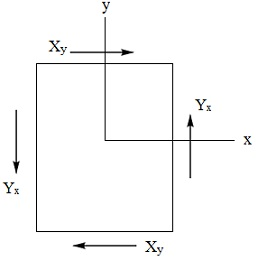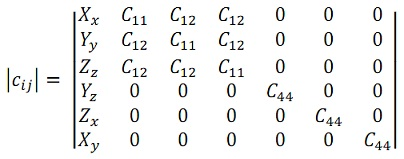#### Elastic Constants of Crystals - I, Physics tutorial

Introduction:

The study of the elastic behavior of solids is extremely significant in the basic and technical researches. In technology, it would state us regarding the strength of the materials. In basic research, it is of interest because of the insight it gives in to the nature of binding forces in solids. They are as well of significance for the thermal properties of solids.

Definition:

Elasticity is basically the study of the capability of crystals to incorporate changes or adapt to new conditions easily.

Analysis of elastic strains and stresses:

The local elastic strain of a body might be specified through six numbers. If α, β, γ are the angles between the unit cell axes a, b, c, the strain might be specified through the changes Δα, Δβ, Δγ; Δa, Δb, Δc resultant from the deformation. This is an excellent physical specification of strain, however for non-orthogonal axes it leads to the arithmetical complications. The strain might be specified in terms of the six components exx, eyy, ezz, exy, eyz, ezx which are stated below. We assume that three orthogonal axes f, g, h of unit length are embedded securely in the unstrained solid, as described in the figure below. We assume that after a small uniform deformation has taken place the axes that we now label, f', g', h' are distorted in orientation and in length, in such a way that having the similar atom as origin we might represent.f' = (1 + εxx)f + εxyg + εxzh;

g' = εyxf + (1 + εyy)g + εyzh;

h' = εzxf + εzyg + (1 + εzz)h;

The fractional changes of length of f, g and h. axes are εxx, εyy, εzz correspondingly, to the first order. We state the strain components exx, eyy, ezz through the relations:

exx = εxx; eyy = εyy; ezz = εzz;

The strain components exy, eyz, ezx might be stated as the changes in angle between the axes, in such a way that to the first order:

exy = f'.g' = εyx + εxy;

eyz = g'.h' = εzy + εyz;

ezx = h'.f' = εzx + εxz;

This completes the statement of the six strain components. A deformation is uniform when the values of the strain components are independent of the choice of origin.

We note that just rotating the axes doesn't change the angle between them, therefore for a pure rotation εyx = -εxy; εzy = -εyz; εzx = -εzx.  Whenever we exclude pure rotations, we might without additional loss of generality take εyx = εxy; εzy = εyz; εzx = εzx. So that in terms of the strain components we encompass:

f' - f = exx + 1/2 exyg + 1/2 ezxh;

g' - g = 1/2 exyf + eyyg + 1/2 eyzh;

h' - h = 1/2 ezxf + 1/2 eyzg + ezzh;

We suppose beneath a deformation that is substantially uniform close to the origin a particle originally at the position:

r = xf + yg + zh

Subsequent to deformation the particle is at:

r' = xf' + yg' + zh'

In such a way that the displacement is represented by:

ρ = r' - r = x(f' - f) + y(g' - g) + z(h' - h)

If we represent the displacement as:

ρ = uf + vg + wh

The expressions for the strain components are as follows:

exx = ∂u/∂x; eyy = ∂v/∂y; ezz = ∂w/∂z;

exy = ∂v/∂x + ∂u/∂y; eyz = ∂w/∂y + ∂v/∂z; ezx = ∂u/∂z + ∂w/∂x;

We have represented derivatives for application to non-uniform strain. The above expressions are often employed in the literature to state the strain components. Occasionally statements of exy, eyz and ezx are given that differ by a factor 1/2 from those given here. For a uniform deformation the displacement 'ρ' consists of the components.

u = exxx + 1/2 exyy + 1/2 ezxz;

v = 1/2 exyx + eyyy + 1/2 eyzz;

w = 1/2 ezxx + 1/2 eyzy + ezzz;

Dilation:

The fractional increment of volume caused through a deformation is termed as the dilation. The unit cube of edges f, g and h, after deformation consists of a volume:

V' = f'.g' X h' ≈ 1 + exx + eyy + ezz

Here squares and products of the strain components are neglected. Therefore the dilation is:

δ = ΔV/V' = exx + eyy + ezz

Shearing strain:

We might interpret the strain components of the kind:

exy = ∂v/∂x + ∂u/∂y

as build up of two simple shears. In one of the shears, planes of the material normal to the x-axis slide in the y-direction; in the other shear, planes normal to y slide in the x-direction.

Stress Components:The force acting on the unit area in the solid is stated as the stress. There are nine stress components: Xx, Xy, Xz, Yx, Yy, Yz, Zx, Zy, Zz. The capital letter points out the direction of the force, and the subscript points out the normal to the plane to which the force is applied. Therefore the stress component Xx symbolizes a force applied in the x-direction to a unit area of a plane whose normal lies in the x-direction; the stress component Xy symbolizes a force applied in the x-direction to a unit area of a plane whose normal lies in the y-direction.

The number of independent stress components is decreased to six via applying to an elementary cube as shown in the figure above. The condition that the angular acceleration disappear and therefore that the total torque should be zero. It follows that:

Yz = Zy, Zx = Xz, Xy = Yx

And the independent stress components might be taken as Xx, Yy, Zz, Yz, Zx, Xy. The stress components encompass the dimensions of force per unit area or energy per unit volume that the strain components are dimensionless.

Elastic Compliance and Stiffness Constants:

Hooke's law defines that for the small deformations the strain is proportional to the stress, in such a way that the strain components are linear functions of the stress components:

exx = s11Xx + s12Yy + s13Zz + s14Yz + s15Zx + s16Xy;

eyy = s21Xx + s22Yy + s23Zz + s24Yz + s25Zx + s26Xy;

ezz = s31Xx + s32Yy + s33Zz + s34Yz + s35Zx + s36Xy;

eyz = s41Xx + s42Yy + s43Zz + s44Yz + s45Zx + s46Xy;

ezx = s51Xx + s52Yy + s53Zz + s54Yz + s55Zx + s56Xy;

exy = s61Xx + s62Yy + s63Yz + s64Yz + s65Zx + s66Xy;

On the contrary, the stress components are linear functions of the strain components:

Xx = c11exx + c12eyy + c13ezz + c14eyz + c15ezx + c16exy;

Yy = c21exx + s22eyy + c23ezz + c24eyz + c25ezx + c26exy;

Zz = c31exx + c32eyy + c33ezz + c34eyz + c35ezx + c36exy;

Yz = c41exx + c42eyy + c43ezz + c44eyz + c45ezx + c46exy;

Zx = c51exx + c52eyy + c53ezz + c54eyz + c55ezx + c56exy;

Xy = c61exx + c62eyy + c63ezz + c64eyz + c65ezx + c66exy;

The quantities s11.... s12 are termed as the elastic constants or elastic compliance constants; the quantities c11.... c11 are termed as the elastic stiffness constants or moduli of elasticity. Other names are as well current. The S's and C's encompass the dimension of area per unit force or volume per unit energy and force per unit area or energy per unit volume correspondingly.

Energy Density:

We compute the increment of work 'δW' done via the stress system in straining a small cube of side 'L', with the origin at one corner of the cube and the coordinate axes parallel to the cube edges. We encompass:

δW = F.δρ

Here 'F' is the applied force and

δρ = fδu + gδv + hδw

is the displacement. If X, Y, Z represent the components of 'F' per unit area, then

δw = L2(Xδu + Yδv + Zδw)

We note that the displacement of the three cube faces having the origin is zero, in such a way that the forces all act at a distance 'L' from the origin. Now by the statement of the strain components:

δu = L(δexx + 1/2 δexy + 1/2 δezx) and so on, in such a way that:

δW = L3(Xxδexx + Yyδeyy + Zzzδezz + Yzδeyz + Zzxδezx + Xyδexy)

The increment δU of the elastic energy per unit volume is represented by:

δU = Xxδexx + Yyδeyy + Zzδezz + Yzδeyz + Zxδezx + Xyδexy

We have δU/δexx = Xx and δU/δeyy = Yy and on further differentiation:

δXx/δeyy = δYy/δexx

From the above, we get the relation:

c12 = c21

And in general we encompass:

cij = cji

Providing fifteen relations between the thirty non-diagonal terms of the matrix of the Cs. The thirty-six elastic stiffness constants are in this way decreased to twenty-one coefficients. Identical relations hold among the elastic compliances. The matrix of the Cs or S's is thus symmetrical.

Cubic crystal:

The number of independent elastic stiffness constants is generally decreased if the crystal has symmetry elements, and in the significant case of cubic crystals there are just three independent stiffness constants, as we now represent. We assume that the coordinate axes are selected parallel to the cube edges. From the equation above which represents stress components are linear functions of the strain components, we have:

c14 = c15 = c16 = c24 = c25 = c26 = c34 = c35 = c36 = 0

As the stress should not be modified by reversing the direction of one of the other coordinate axes. As the axes are equivalent, we as well have:

c11 = c22 = c33,

And c12 = c13 = c21 = c23 = c31 = c32,

We also have:

c44 = c15 = c66

by equivalence of the axes, and the other constants all disappear due to the reason of their behavior on reversing the direction of one or other axis. The array of values of the elastic stiffness constant is thus decreased for a cubic crystal to the matrix illustrated below:This is readily observed that for a cubic crystal:

U = (1/2) c11(e2xx + e2yy + e2zz) + c12(eyyezz + ezzexx + exxeyy) + (1/2) c44(e2yz + e2zx + e2xy)

satisfies the equation for the elastic energy density function.

For illustration, δU/δeyy = c11eyy + c12ezz + c12exx = Yy

By using the equation of cubic crystal, for cubic crystals the compliance and stiffness constants are associated by:

C11 = [(s11 + s12)/(s11 - s12)(s11 + 2s12)];

C12 = [(-s12)/(s11 - s12)(s11 - 2s12)]

C14 = 1/s44

A general evaluation of elastic constant data and of relationships between different coefficients for the crystal classes has been illustrated through Hearmon in the year 1946).

Tutorsglobe: A way to secure high grade in your curriculum (Online Tutoring)